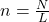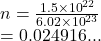## 1 point How many moles of magnesium is 1.50 x 10^22 atoms of magnesium?

Question

1 point
How many moles of magnesium is 1.50 x 10^22 atoms of magnesium?

in progress 0
6 months 2021-08-25T00:05:03+00:00 1 Answers 3 views 0

## 0.025 moles

Explanation:

To find the number of moles in a substance given it’s number of entities we use the formulawhere n is the number of moles

N is the number of entities

L is the Avogadro’s constant which is

6.02 × 10²³ entities

We haveWe have the final answer as

### 0.025 moles

Hope this helps you